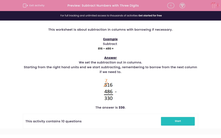# Subtract Numbers with Three Digits

In this worksheet, students subtract numbers with three digits using the column method with borrowing if necessary.This content is premium and exclusive to EdPlace subscribers.Key stage:  KS 2

Curriculum topic:   Maths and Numerical Reasoning

Curriculum subtopic:   Mixed Problems

Difficulty level:#### Worksheet Overview

This worksheet is about subtraction in columns with borrowing if necessary.

Example

Subtract

816 – 486 =

We set the subtraction out in columns.

Starting from the right hand units end we start subtracting, remembering to borrow from the next column if we need to.